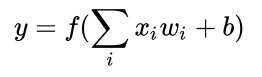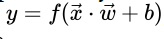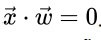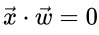# Explain what a neuron is, in terms of Neural Network in Machine Learning.

A neuron is a mathematical function that takes one or more values as input and outputs a ingle numerical value −

It can be defined as follows −Here, ‘f’ refers to the function.

• We first computed the weighted sum of the inputs xi and the weights wi

• The weight wi is also known as the activation value or activation function.

• The input xi can be a numerical value that represents the input data or it can be an output from other neurons if the neuron belong to a neural network.

• The weight wi is a numerical value that can be used to represent the strength of the inputs or the strength of the connection links between the neurons.

• The weight ‘b’ is a special value known as bias whose input is always 1.

• The bias ‘b’ is used to allow the hyperplane to shift away from the centre of the coordinate system.

• If bias isn’t used, the neuron will have limited representation power.

The result of the weighted sum is passed as an input to the activation function f, which is also known as the transfer function.

There are different types of activation functions, but all of them have to satisfy the requirement of being non-linear.

An important observation is that the neuron is similar to logistic regression and the perceptron.

Neuron can be understood a generalized version of logistic regression and perceptron algorithms. If the logistic function or step function is used as an activation function, the neuron can be used as logistic regression or perceptron respectively.

In addition to this, if we don't use any activation function, the neuron becomes a linear regression problem.

The activation value previously defined can be interpreted as the dot product of the vector w and the vector x.The vector x would be perpendicular to the weight vector w, if −Hence, all vectors x,that define a hyperplane in the feature space Rn , where n is the dimension of x.

Let us understand the above statement in simple terms − If the activation function is f(x) = x and we have a single input value x, the output of the neuron becomes y = wx + b. This is the linear equation.

This indicates that in one dimensional input space, the neuron basically gives the equation of a line, i.e defines a line.

If we visualize the same for two or more inputs, the neuron defines a plane. If the input dimensions are arbitrary in number, the neuron defines a hyperplane.

The perceptron (neuron) works only with linearly separable classes, and this is because it defines a hyperplane. To overcome this limitation, the neurons are organized into a neural network.